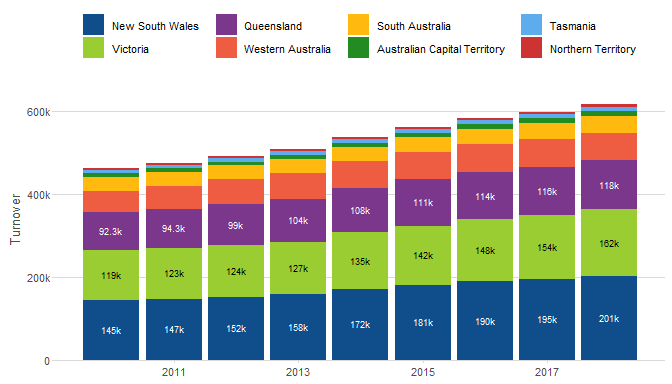# Overview

Use ezplot to quickly create presentation-ready charts that are also useful for exploratory data analysis. By default, ezplot functions aggregate multiple values of y for repeated categories of x, group, facet_y and facet_x.

library(ezplot)
library(tsibble)
library(tsibbledata)
suppressPackageStartupMessages(library(lubridate))
library(ggplot2)
library(grid)

### line_plot

Weekly aggregation:

line_plot(ansett, x = "Week", y = "Passengers")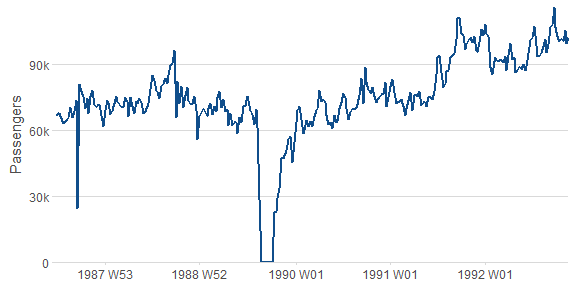Add grouping:

line_plot(ansett, x = "Week", y = "Passengers", group = "Class")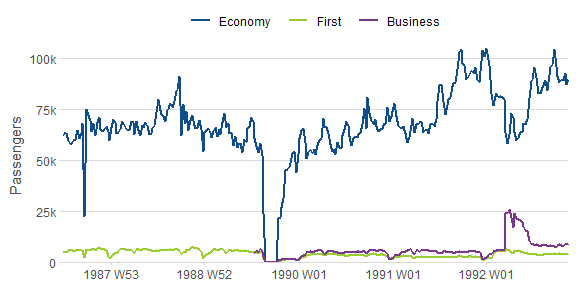Add faceting:

line_plot(ansett, x = "Week", y = "Passengers",
group = "Class", facet_x = "Airports",
facet_scales = "free_y", size = 10) +
theme(axis.text.x = element_text(angle = 90, vjust = 0.38, hjust = 1))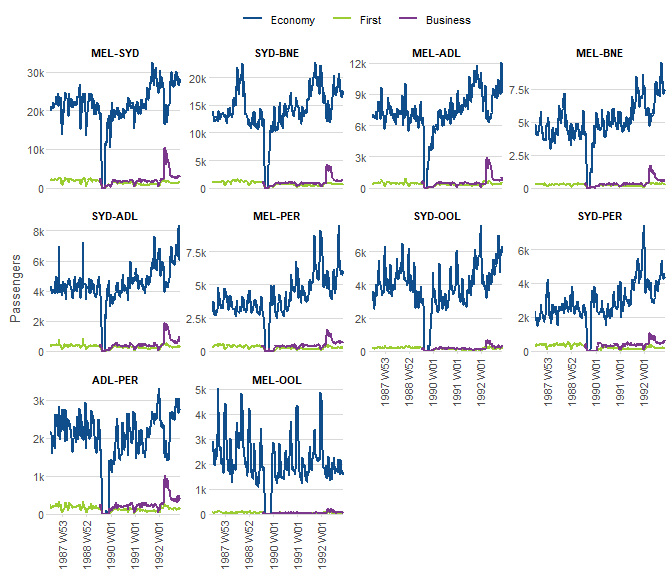Plot YOY comparisons:

line_plot(gafa_stock, "Date", c("Closing Stock Price" = "Close"),
facet_y = "Symbol",
facet_scales = "free_y",
yoy = TRUE,
labels = function(x) ez_labels(x, prepend = "\$"))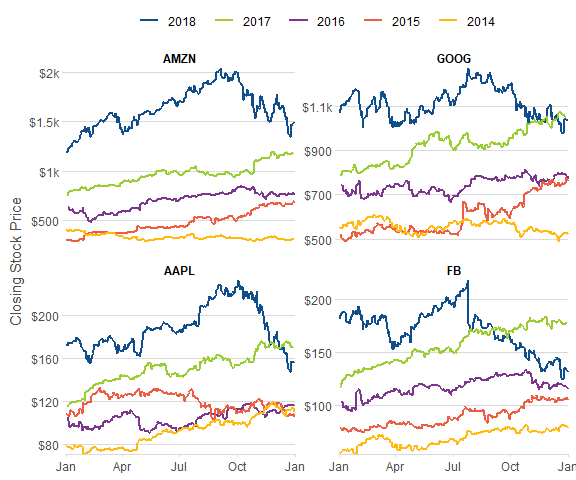Plot multiple numeric columns:

line_plot(hh_budget,
"Year",
c("DI", "Expenditure", "Savings"),
facet_x = "Country") +
theme(panel.spacing.x = unit(1, "lines")) +
ylab(NULL)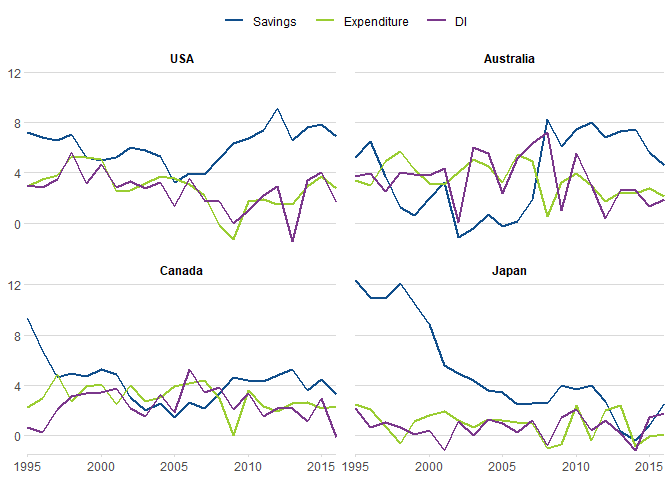### area_plot

Weekly aggregation:

area_plot(ansett, x = "as.Date(Week)", y = "Passengers")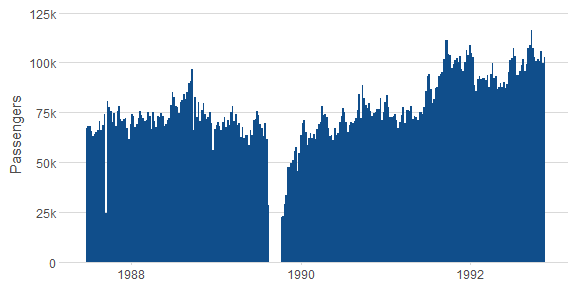Add grouping:

area_plot(ansett, x = "as.Date(Week)",
y = c("Weekly Passengers" = "Passengers"),
"Class")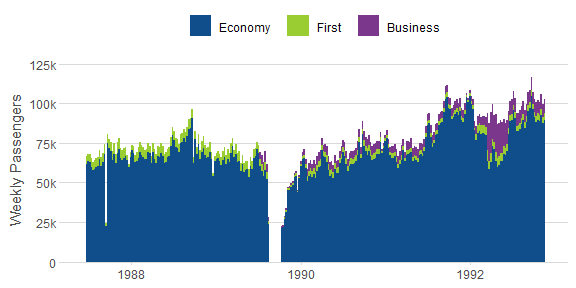Add faceting:

area_plot(ansett,
"year(Week) + (month(Week) - 1) / 12",
y = c("Monthly Passengers" = "Passengers"),
group = "substr(Airports, 5, 7)",
facet_x = "substr(Airports, 1, 3)", facet_y = "Class",
facet_scales = "free_y") +
theme(axis.text.x = element_text(angle = 90, vjust = 0.38, hjust = 1))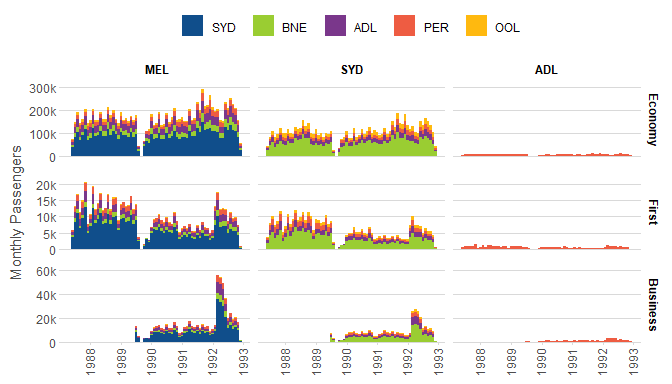### bar_plot

Yearly aggregation

bar_plot(subset(aus_retail, year(Month) >= 2010),
x = "year(Month)",
y = "Turnover")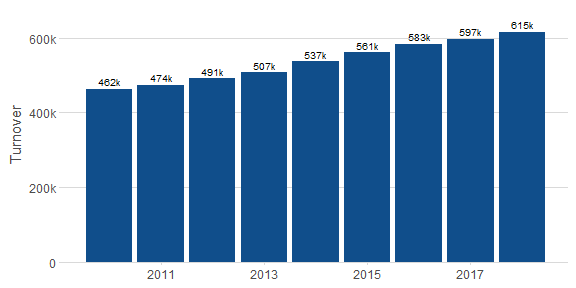With grouping:

bar_plot(subset(aus_retail, year(Month) >= 2010),
x = "year(Month)",
y = "Turnover",
group = "State",
size = 10)